Next: Equipartition Theorem Up: Applications of Statistical Thermodynamics Previous: Gibb's Paradox

# General Paramagnetism

In Section 7.3, we considered the special case of paramagnetism in which all the atoms that make up the substance under investigation possess spin 1/2. Let us now discuss the general case.

Consider a system consisting of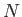non-interacting atoms in a substance held at absolute temperature, and placed in an external magnetic field,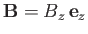, that points in the-direction. The magnetic energy of a given atom is written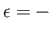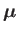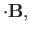(7.91)

where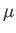is the atom's magnetic moment. Now, the magnetic moment of an atom is proportional to its total electronic angular momentum,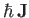. In fact,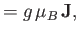(7.92)

where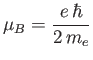(7.93)

is a standard unit of magnetic moment known as the Bohr magneton. Here,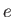is the magnitude of the electron charge, and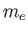the electron mass. Moreover,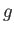is a dimensionless number of order unity that is called the g-factor of the atom.

Combining Equations (7.91) and (7.92), we obtain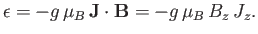(7.94)

Now, according to standard quantum mechanics,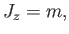(7.95)

where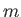is an integer that can take on all values between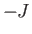and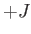. Here,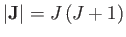, where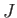is a positive number that can either take integer or half-integer values. Thus, there are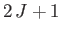allowed values of, corresponding to different possible projections of the angular momentum vector along the-direction. It follows from Equation (7.94) that the possible magnetic energies of an atom are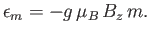(7.96)

For example, if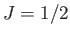(corresponding to a spin-1/2 system) then there are only two possible energies, corresponding to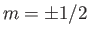. This was the situation considered in Section 7.3. (To be more exact, a spin-1/2 system corresponds toand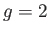.)

The probability,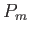, that an atom is in a state labeledis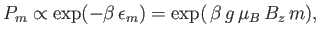(7.97)

where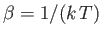. According to Equation (7.92), the corresponding-component of the magnetic moment is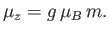(7.98)

Hence, the mean-component of the magnetic moment is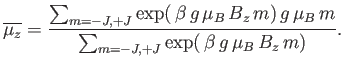(7.99)

The numerator in the previous expression is conveniently expressed as a derivative with respect to the external parameter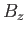: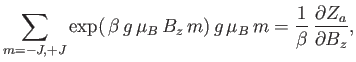(7.100)

where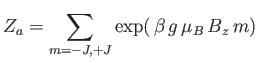(7.101)

is the partition function of a single atom. Thus, Equation (7.99) becomes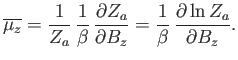(7.102)

In order to calculate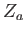, it is convenient to define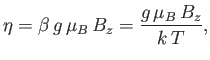(7.103)

which is a dimensionless parameter that measures the ratio of the magnetic energy,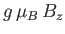, that acts to align the atomic magnetic moments parallel to the external magnetic field, to the thermal energy,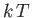, that acts to keep the magnetic moment randomly orientated. Thus, Equation (7.101) becomes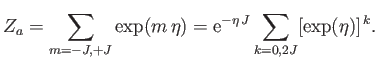(7.104)

The previous geometric series can be summed to give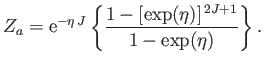(7.105)

(See Exercise 1.) Multiplying the numerator and denominator by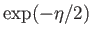, we obtain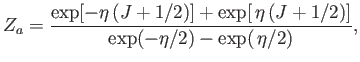(7.106)

or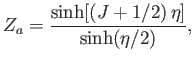(7.107)

where the hyperbolic sine is defined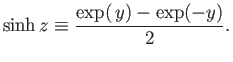(7.108)

Thus,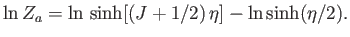(7.109)

According to Equations (7.102) and (7.103),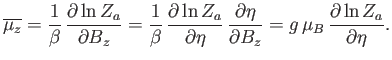(7.110)

Hence,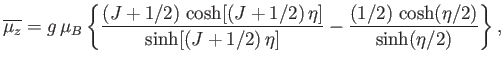(7.111)

or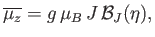(7.112)

where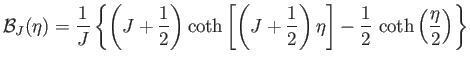(7.113)

is known as the Brillouin function.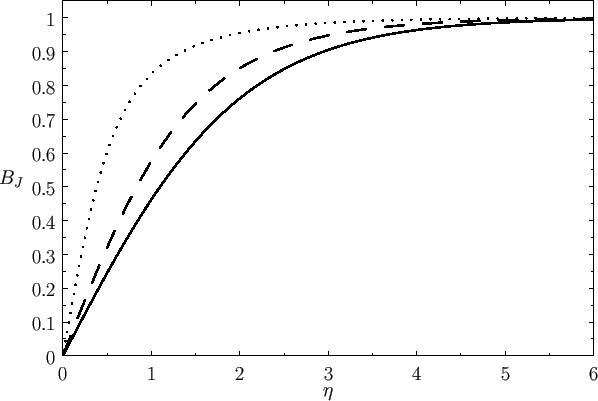The hyperbolic cotangent is defined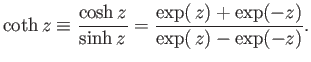(7.114)

For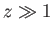, we have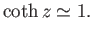(7.115)

On the other hand, for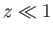,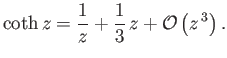(7.116)

Thus, it follows that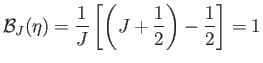(7.117)

when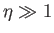. On the other hand, when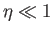, we get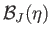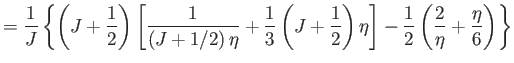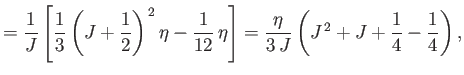(7.118)

which reduces to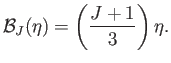(7.119)

Figure 7.1 shows howdepends on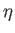for various values of.If there are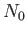atoms per unit volume then the mean magnetic moment per unit volume (or magnetization) becomes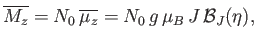(7.120)

where use has been made of Equation (7.112). Ifthen Equation (7.119) implies that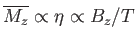. This relation can be written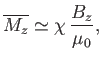(7.121)

where the dimensionless constant of proportionality,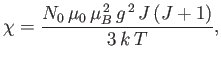(7.122)

is known as the magnetic susceptibility. Thus,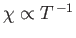--a result that is called Curie's law. (See Section 7.3.) Ifthen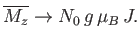(7.123)

This corresponds to magnetic saturation--a situation in which each atom has the maximum component of the magnetic moment parallel to the magnetic field,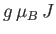, that it can possibly have.

Figure 7.2 compares the experimental and theoretical magnetization versus field-strength curves for three different paramagnetic substances containing atoms of total angular momentum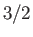,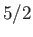, and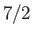particles, showing excellent agreement between theory and experiment. Note that, in all cases, the magnetization is proportional to the magnetic field-strength at small field-strengths, but saturates at some constant value as the field-strength increases.

The previous analysis completely neglects any interaction between the spins of neighboring atoms or molecules. It turns out that this is a fairly good approximation for paramagnetic substances. However, for ferromagnetic substances, in which the spins of neighboring atoms interact very strongly, this approximation breaks down completely. (See Section 7.17.) Thus, the previous analysis does not apply to ferromagnetic substances.Next: Equipartition Theorem Up: Applications of Statistical Thermodynamics Previous: Gibb's Paradox
Richard Fitzpatrick 2016-01-25